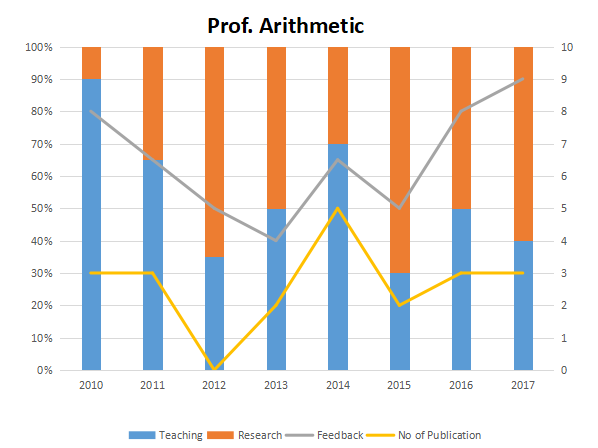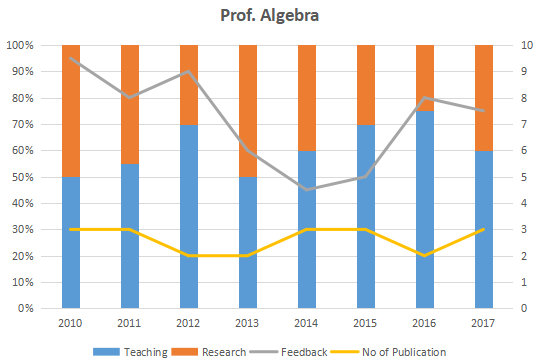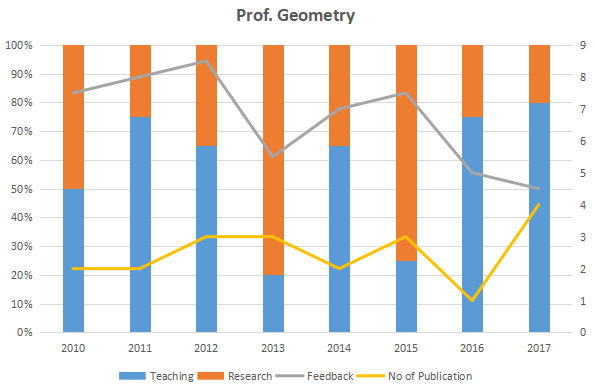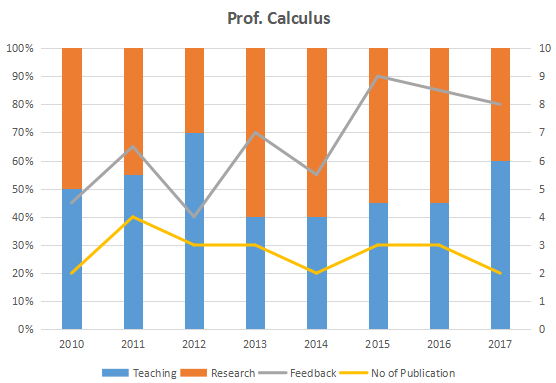### XAT 2018 Question 74

Instructions

The graph below represent the performance of four professors, across years. measured on four variables: Percentage of time spent on research, teaching, feedback on (a scale of 10. right-hand side) and number of publications (right-hand side). Assume that the cumulative time spent per year on research and teaching activities are sane for all four professors and each of them taught only one course of 90 classroom hours.Question 74

# Research efficiency is the ratio of cumulative number of publication for a period of 3 years to the cumulative number of hours spent on research activity in those 3 years. Which of the following professors is the least efficient researcher for the period 2015 to 2017?

Solution

Research efficiency = (total number of publications)/(total time spent in research)

Prof. Arithmetic: RE = (8) / [90*(0.7 + 0.5 + 0.6)] = 8 / (18 * 9)

Prof. Algebra: RE = (8) / [90*(0.3 + 0.4 + 0.25)] = 8 / (9.5 * 9)

Prof. Geometry: RE = (8) / [90*(0.75 + 0.25 + 2)] = 8 / (12 * 9)

Prof. Calculus: RE = (8) / [90*(0.55 + 0.55 + 0.4)] = 8 / (15 * 9)

Thus, we can observe that Prof. Arithmetic has the lowest efficiency.

Hence, option A is the answer.